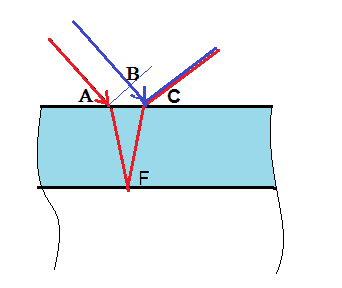# Maximum/minimum reflection | Thin-film interference

## Homework Statement

A transparent film( n = 1.45) is placed on a glass surface( n = 1.14) and is illuminated by light ( incidence angle α = 60°. What is the minimum thickness d1 of film such that reflection of light with λ is minimized, while if the thickness is d2 then with the same λ the reflection is maximized?

## Homework Equations

2dcosβ = (m - .5)λ/n
β = 36.6°
m = 0,1,2,3...
d - film thickness
λ = light wavelength in vacuum (air)

## The Attempt at a Solution

I am not good at physics, but I am supposed to do my homework. So, I pointed out that I am dealing with constructive interference and found the equation I showed above(used "Physics for Scientists and Engineers" A.Serway for that).
My first problem is to find λ. I tried to substitute d2 in the equation and then I understood that I don't know what value of m I should use. For example, I used m = 1 and got λ = 648×10^(-9). But in this case, how am I supposed to find d1? If I use the same equaton, the same m and λ I will get d1 = d2. I've got completely confused by these maximum and minimum reflections

lekh2003
Gold Member
I don't seem to understand the question. Is there some kind of relation between d1 and d2? The way I see it, they look like they could be anything. What are their values? What are the values you need to find? Wavelength or distance?

phyzguy
You don't need to find λ. You are supposed to take λ as given, and then find d1 and d2. You answers for d1 and d2 will contain λ, so they will both be functions of λ.

Are you sure about the formula: 2dcosβ = (m - .5)λ/n
If the light ray travels a perpendicular distance d within the film then
wouldn"t the actual ray travel a distance L = d / cosβ ?

ehild
Homework Helper
If I use the same equation, the same m and λ I will get d1 = d2. I've got completely confused by these maximum and minimum reflections
You have different equation for interference maximums and minimums. For a maximum, 2dncosβ + λ/2= mλ. For a minimum, the interference is destructive, 2dncosβ + λ/2 = (2m+1)λ/2 ---> 2dncosβ=mλ.

•lekh2003
ehild
Homework Helper
Are you sure about the formula: 2dcosβ = (m - .5)λ/n
If the light ray travels a perpendicular distance d within the film then
wouldn"t the actual ray travel a distance L = d / cosβ ?
Actually, the ray travels 2d/cosβ in the layer. And the optical path has to be taken into account when calculating interference, which is the geometric path multiplied with the refractive index. The other ray it interferes with, reflected directly at the interface, also travels some distance from their common phase plane AB. The optical path difference between the blue and red rays is 2 n AF -BC.#### Attachments

Actually, the ray travels 2d/cosβ in the layer. And the optical path has to be taken into account when calculating interference, which is the geometric path multiplied with the refractive index. The other ray it interferes with, reflected directly at the interface, also travels some distance from their common phase plane AB. The optical path difference between the blue and red rays is 2 n AF -BC.
View attachment 214923
Agreed!
The phase difference in the plane wave, as you specified
2 n d / cos β - 2 d tan β sin α does simplify to 2 n d cos β
since sin α = n sin β